# Virtual Localization for Mesh Network Routing

Nick Moore / Ahmet Sekercioglu / Gregory K Egan

## Addendum: 200 Node Mesh

Typically, mesh routing algorithms are tested on a network with nodes placed completely at random. Randomly assigning the locations of nodes is inefficient, with large numbers of nodes required to provide complete coverage. A high density of nodes is needed if the network is not to be disjoint.

Instead, for this paper we have placed nodes randomly within the restriction that the minimum distance between any two nodes is half the range, and that every node is placed within range of at least one neighbour. This ensures a non-disjoint network with a limit on the density of nodes and a limit on the number of neighbours of any given node. This kind of placement would be typical where it is possible to select the approximate position of a node but the exact position depends on other factors such as the terrain. The Virtual Localization algorithm can then be used to find an efficient routing map of the network.

Figure 2 shows a network constructed in this manner, and illustrates the process of determining virtual locations for each node in the network. The area of interest is one square kilometre, and the communication range is 100 metres.

### Figure 2A: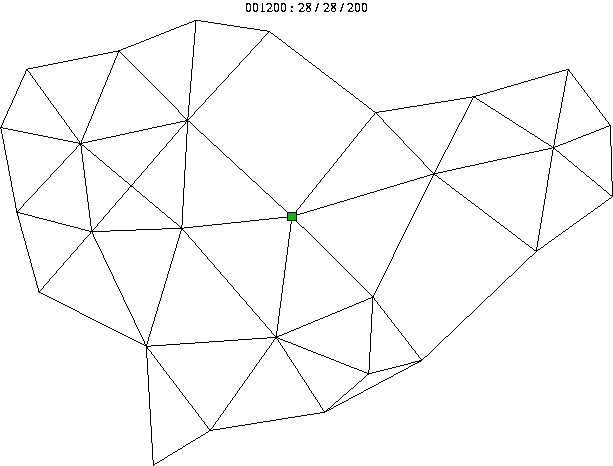### Figure 2B: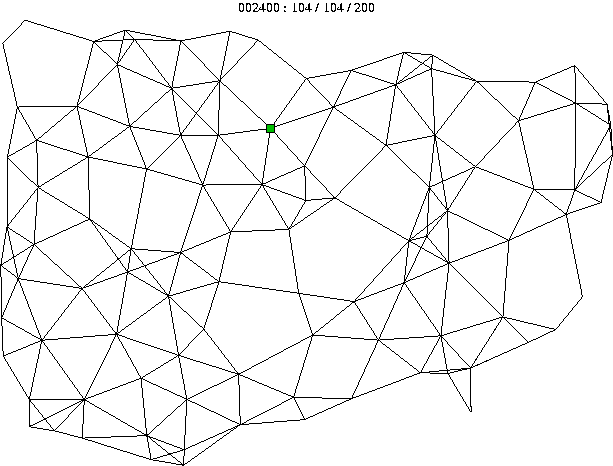### Figure 2C: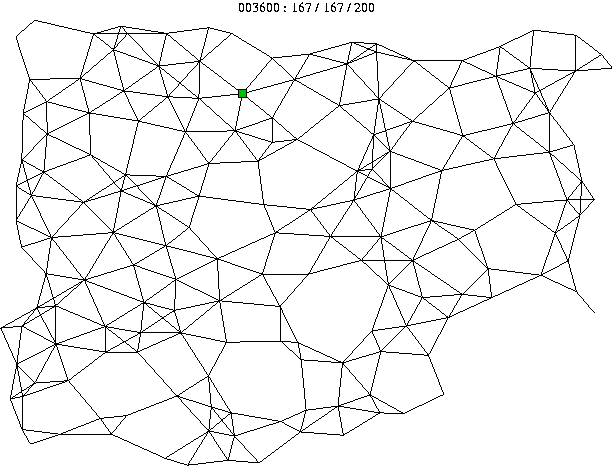### Figure 2D: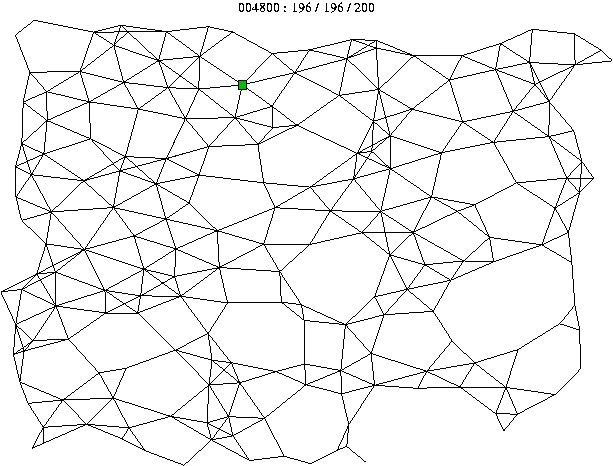### Figure 2E: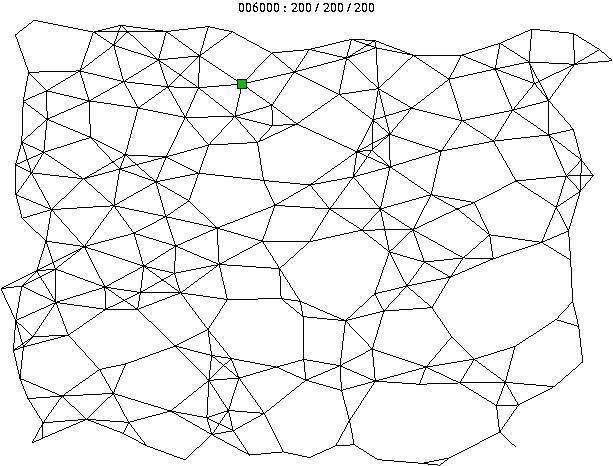### Figure 2F:Figure 2: This diagram shows a series of snapshots at A: 1200, B: 2400, C: 3600, D: 4800 and E: 6000 milliseconds, as the nodes progressively discover their virtual locations; %By 6000 ms, the entire network has been virtually mapped, F: a map of the original network layout for comparison purposes. The root node is marked.